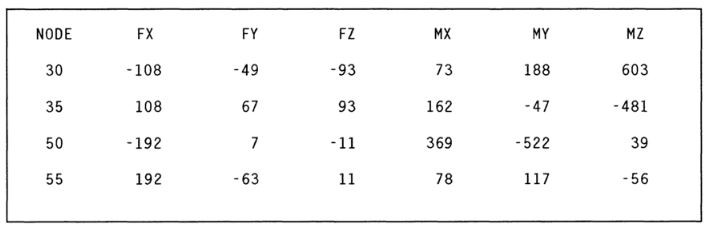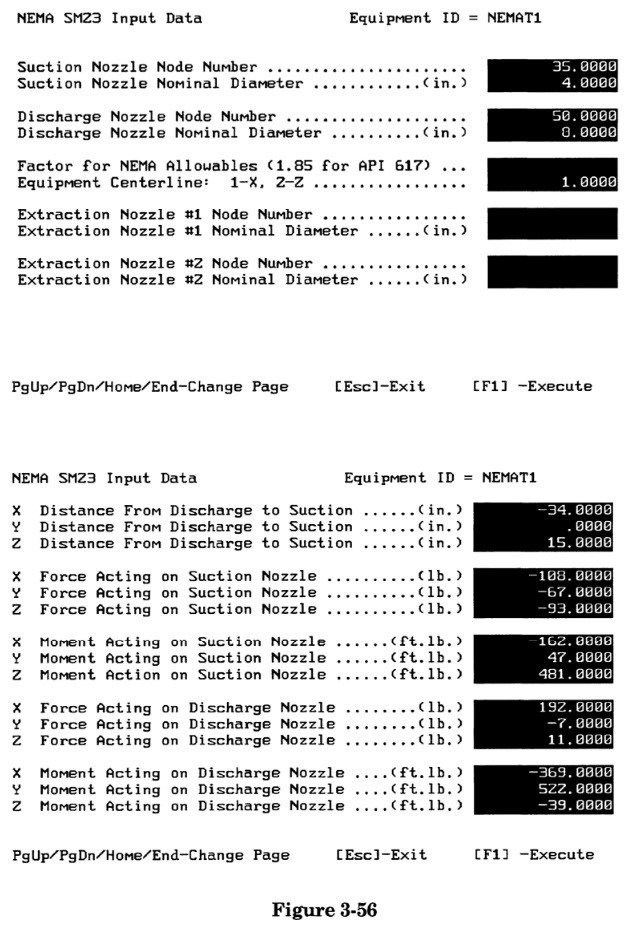Search

# 3.4.1.1 NEMA SM23 Standard for Steam Turbines

NEMA Standard SM23 requires that steam turbines be evaluated by two sets of simple force / moment calculations. The two types of computations are used to satisfy:

1. individual nozzle allowables

2. cumulative equipment allowables

First, the loads on each individual suction, discharge, and extraction nozzle must satisfy the equation:

3F + M < 500 D Where:

F = resultant force on the particular nozzle, lb

M = resultant force on the particular nozzle, ft-lb D = nominal pipe size of the connection, in

If the loads on the individual nozzles are acceptable, the cumulative load on the equipment from all nozzles must be checked next. Cumulative equipment allowables require that forces and moments on all connections be resolved at the discharge nozzle; the absolute magnitude of each of the force and moment components must then fall below a multiple of Dc, as:

Fx <= 50 Dc Fy <= 125 Dc Fz <= 100 Dc Mx <= 250 Dc My <= 125 Dc Mz <= 125 Dc Fc + Mc/2 <= 125 Dc

Where:

Fx = total X-force (from all nozzles) on the equipment (where the X-axis is defined as being parallel to the equipment centerline), lb

Fy = total Y-force (from all nozzles) on the equipment (where the Y-axis is coincident with the direction of gravity), lb

Fz = total Z-force (from all nozzles) on the equipment (where the Z-axis is defined by the right hand rule form the other two), lb

Fc = total resultant force acting on the equipment, lb

Mx = total X-moment (from forces and moments on all nozzles) acting on the equipment, resolved about the discharge nozzle, ft-lb

My = total Y-moment (from forces and moments on all nozzles) acting on the equipment, resolved about the discharge nozzle, ft-lb

Mz =total Z-moment (from forces and moments on all nozzles) acting on the equipment, resolved about the discharge nozzle, ft-lb

Mc = total resultant moment acting on the equipment, resolved about the dis charge nozzle, ft-lb

Dc = diameter of an opening whose area is equal to the sum of the areas of all individual equipment nozzles, in

An example of a NEMA SM23 analysis is shown in Figure 3-55. In this example, the turbine is modeled as a series of rigid elements, so both the discharge and suction nozzle loads appear on the same pipe stress analysis output.The forces and moments acting on the elements connected to the turbine (excerpted from the CAESAR II output for this piping system) are shown below:Since the sign conventions of these forces is that of forces acting on the elements, it is necessary to reverse the signs to get the forces and moments acting on the turbine nozzles at points 35 and 50, or:When running the NEMA portion of the ROT program, there are two input spreadsheets upon which descriptive and load data must be entered, which in this case would be filled out as shown in Figure 3-56.The program then proceeds to check the loading on each nozzle, and then resolves the loads about the discharge and checks the cumulative loading on an equivalent nozzle, as described above. The two page NEMA output report for this particular example is shown in Figure 3-57.The results first show that the discharge nozzle passed (only reaching 30.4% of the allowable), the suction nozzle passed (reaching 49% of the allowable), and the cumulative load passed, with the highest summation load (Z-moment) reaching only 56% of the allowable. If the turbine had failed, the symbol **FAILED** would have appeared in the "STATUS" column next to the load combination that was excessive.